# Funnel and Pyramid in WPF Charts (SfChart)

30 Jul 20216 minutes to read

## Pyramid Chart

PyramidSeries has the form of a triangle with lines dividing it into sections and each section has a different width. Depending on the Y co-ordinates, this width indicates a level of hierarchy among other categories.

``````<chart:PyramidSeries XBindingPath="Category"

ItemsSource="{Binding Tax}"

YBindingPath="Percentage"/>``````
``````PyramidSeries series = new PyramidSeries()
{

ItemsSource = new ViewModel().Tax,

XBindingPath = "Category",

YBindingPath = "Percentage"

};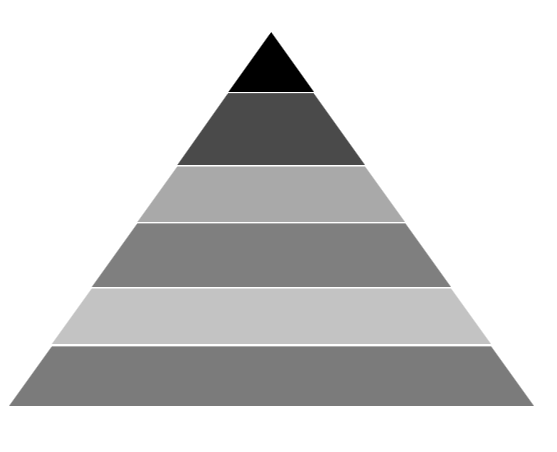The `PyramidMode` is used to define the rendering mode such as `Surface` or `Linear` pyramid segments.

PyramidMode as Surface

``````<chart:PyramidSeries XBindingPath="Category"

PyramidMode="Surface"

ItemsSource="{Binding Tax}"

YBindingPath="Percentage"/>``````
``````PyramidSeries series = new PyramidSeries()
{

ItemsSource = new ViewModel().Tax,

XBindingPath = "Category",

YBindingPath = "Percentage",

PyramidMode = ChartPyramidMode.Surface

};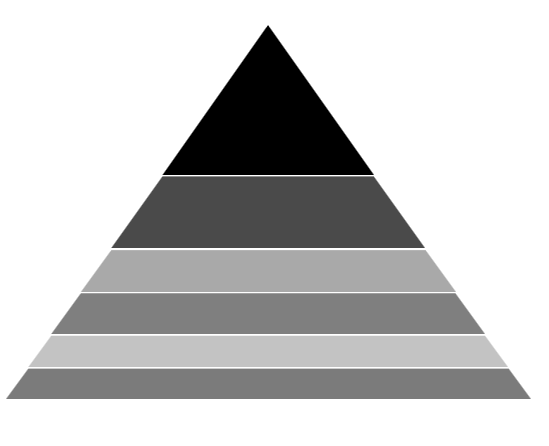PyramidMode as Linear

``````<chart:PyramidSeries XBindingPath="Category"

PyramidMode="Linear"

ItemsSource="{Binding Tax}"

YBindingPath="Percentage"/>``````
``````PyramidSeries series = new PyramidSeries()
{

ItemsSource = new ViewModel().Tax,

XBindingPath = "Category",

YBindingPath = "Percentage",

PyramidMode = ChartPyramidMode.Linear

};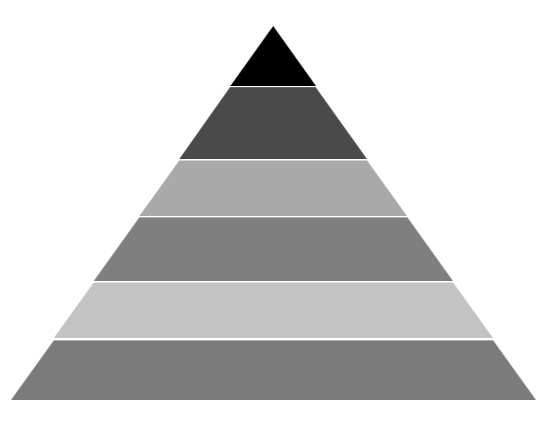NOTE

You can explore our WPF Pyramid Chart feature tour page for its groundbreaking features. You can also explore our WPF Pyramid Chart example to know how to represent time-dependent data, showing trends in data at equal intervals.

## Funnel Chart

FunnelSeries is similar to PyramidSeries, displays data in a funnel shape that equals to 100% when totaled. It is a single series, representing data as portions of 100% and does not use any axes.

The following code example shows how to use the funnel series:

``````<chart:FunnelSeries XBindingPath="Category" ItemsSource="{Binding list}"

YBindingPath="Percentage" />``````
``````FunnelSeries series = new FunnelSeries()
{

ItemsSource = new ViewModel().List,

XBindingPath = "Category",

YBindingPath = "Percentage",

};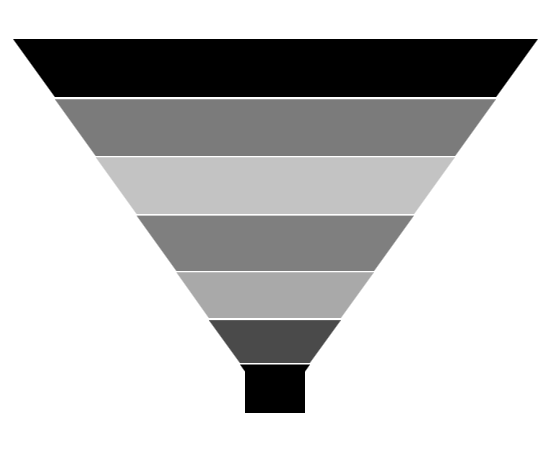### Funnel Mode

The `FunnelMode` defines a rendering mode for the funnel series which define, where to bind your values (to height or width). The following example demonstrates `ValueIsHeight` and `ValueIsWidth` funnel mode:

ValueIsHeight

``````<chart:FunnelSeries XBindingPath="Category" ItemsSource="{Binding list}"

FunnelMode="ValueIsHeight"

YBindingPath="Percentage"/>``````
``````FunnelSeries series = new FunnelSeries()
{

ItemsSource = new ViewModel().List,

XBindingPath = "Category",

YBindingPath = "Percentage",

FunnelMode = ChartFunnelMode.ValueIsHeight

};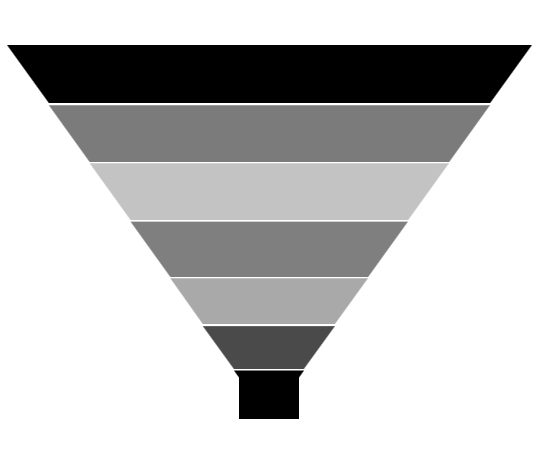ValueIsWidth

``````<chart:FunnelSeries XBindingPath="Category" ItemsSource="{Binding list}"

FunnelMode="ValueIsWidth"

YBindingPath="Percentage" />``````
``````FunnelSeries series = new FunnelSeries()
{

ItemsSource = new ViewModel().List,

XBindingPath = "Category",

YBindingPath = "Percentage",

FunnelMode = ChartFunnelMode.ValueIsWidth

};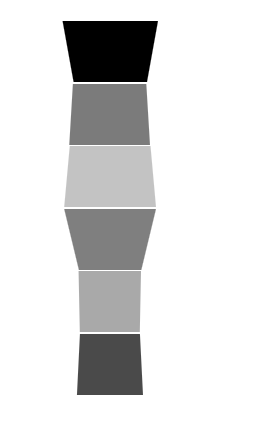### Explode Segments

The following properties are used to explode the individual segments in Funnel and Pyramid.

Explode Offset

``````<chart:FunnelSeries XBindingPath="Category" ItemsSource="{Binding list}"

ExplodeIndex="4"  ExplodeOffset="70" YBindingPath="Percentage">

</chart:FunnelSeries>``````
``````FunnelSeries series = new FunnelSeries()
{

ItemsSource = new ViewModel().List,

XBindingPath = "Category",

YBindingPath = "Percentage",

ExplodeIndex = 4,

ExplodeOffset = 70

};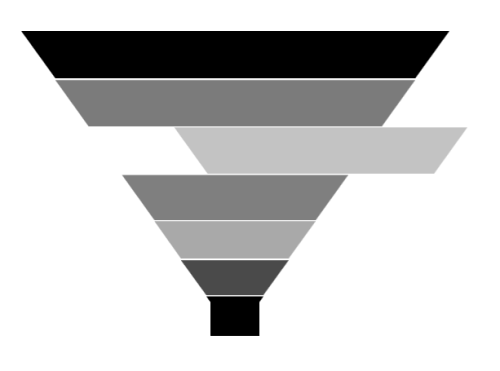Gap Ratio

The gap between each segment using `GapRatio` property as in the following code example.

``````<chart:FunnelSeries XBindingPath="Category" ItemsSource="{Binding list}"

GapRatio="0.5" YBindingPath="Percentage">

</chart:FunnelSeries>``````
``````FunnelSeries series = new FunnelSeries()
{

ItemsSource = new ViewModel().List,

XBindingPath = "Category",

YBindingPath = "Percentage",

GapRatio = 0.5

};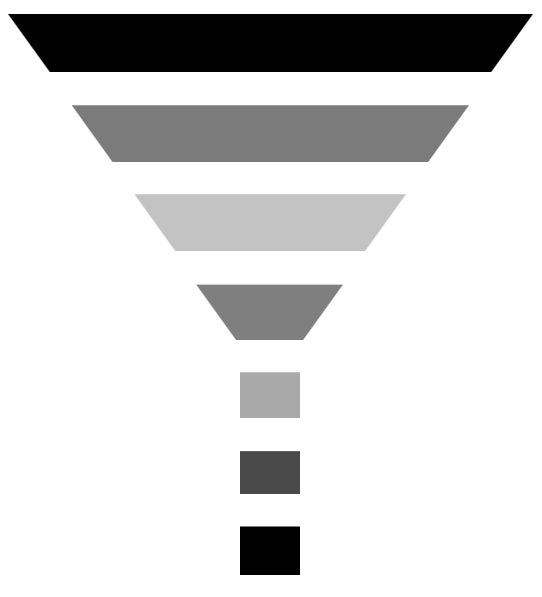`How to add gaps between segments in a pyramid or funnel series`